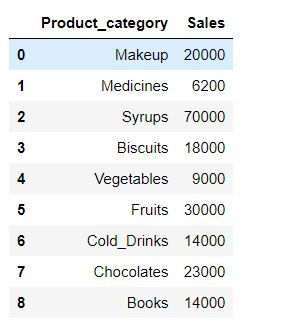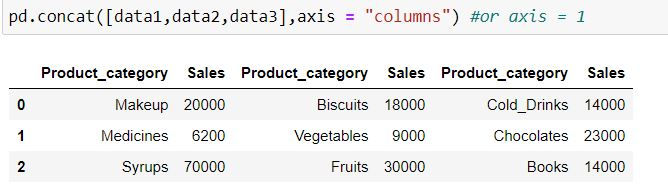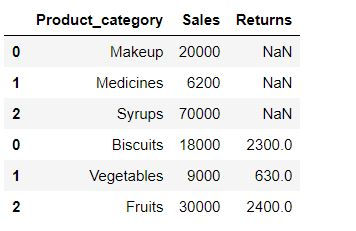top of page
Search
• Ekta Aggarwal

# Concatenating and appending data in Python

When we have various datasets which we want to join one below the other (row-wise) or side by side (as columns) we call it concatenating or appending or binding the dataset.

In this tutorial we shall learn how we can concatenate and append data in Python using pandas' concat and append functions.

Let us firstly import our library pandas

`import pandas as pd`

## pd.concat( )

Using pd.concat( ) we can append our data row-wise as well as column-wise.

Let us consider 3 datasets:

```data1 = pd.DataFrame({'Product_category': ['Makeup','Medicines','Syrups'],
'Sales': [20000,6200,70000]})

data2 = pd.DataFrame({'Product_category': ['Biscuits','Vegetables','Fruits'],
'Sales': [18000,9000,30000]})

data3 = pd.DataFrame({'Product_category': ['Cold_Drinks','Chocolates','Books'],
'Sales': [14000,23000,14000]})```Let us concatenate the data. By default pd.concat( ) appends the data by rows.

`pd.concat([data1,data2,data3])`

Note the indices for the resultant dataset: They are 0, 1 and 2. When another data is appended below it then indices are taken for another dataset.Suppose data1 was for January, data2 for February and data3 for March then we can specify the indices denoting the keys

```pd.concat([data1,data2,data3],ignore_index=False,
keys = ['Jan','Feb','Mar'])```

Notice: Now we have indices and keys corresponding to every data which was used for concatenation.We can make the incides start from 0 and create new indices by specifying ignore_index = True

`pd.concat([data1,data2,data3],ignore_index=True)`

Note that in the resultant data new indices are from 0 to 8.By default pd.concat( ) concatenates data by row. i.e. axis = "index" or axis = 0.

To add columns to the data we define axis = "columns" or axis = 1

`pd.concat([data1,data2,data3],axis = "columns") #or axis = 1`### What happens when we have unequal number of columns?

Let us consider 2 datasets where data1 has 2 columns and data2 has 3 columns:

```data1 = pd.DataFrame({'Product_category': ['Makeup','Medicines','Syrups'],
'Sales': [20000,6200,70000]})

data2 = pd.DataFrame({'Product_category': ['Biscuits','Vegetables','Fruits'],
'Sales': [18000,9000,30000],
"Returns" : [2300,630,2400]})````pd.concat([data1,data2])`On concatenating data1 and data2, since Returns column is unavailable in data1 thus we have missing values for it.

### sort = True

If we define sort = True then the column in the resultant dataset get sorted by their column names in ascending order

`pd.concat([data1,data2],sort =True)`### join = "inner"

To keep only common columns in the result we define join = "inner" i.e. column with missing values would be removed.

`pd.concat([data1,data2],join = 'inner')`## append( ) function

Pandas' append function is used only to append the datasets row-wise. You cannot append them column-wise.

Let us consider following 2 datasets:

```data1 = pd.DataFrame({'Product_category': ['Makeup','Medicines','Syrups'],
'Sales': [20000,6200,70000]})

data2 = pd.DataFrame({'Product_category': ['Biscuits','Vegetables','Fruits'],
'Sales': [18000,9000,30000],
"Returns" : [2300,630,2400]})```append( ) has the provision to ignore the indices like concat( ) function by specifying ignore_index = True.

`data1.append(data2,ignore_index = True)`

append( ) also has the provision for sorting the columns by their column names like concat( ) .

`data1.append(data2,sort= True)`

Note: append( ) always returns all of the columns in both the datasets. You can't specify join = "inner" in append like you could in concat( ) function.

Similarly you cannot specify keys in append( ).

bottom of page